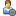Hello Guest it is October 01, 2020, 04:41:46 PM

###Show Posts

This section allows you to view all posts made by this member. Note that you can only see posts made in areas you currently have access to.

### Topics - schweißer

Pages: 1
1
##### G-Code, CAD, and CAM discussions / Sheetcam postprocessor
« on: February 10, 2014, 06:26:38 AM »
Hello,

i need help with postprocessor for sheetcam and Mach3.

This is my postprocessor

ctrl = event:GetTextCtrl()
ctrl:AppendText("Plasma MP1000-THC -ZRef post processor\n")
ctrl:AppendText("Use with floating head (auto touch off) ONLY\n")
ctrl:AppendText("Modal G-codes and coordinates\n")
ctrl:AppendText("Comments enclosed with ( and )\n")
ctrl:AppendText("M03/M05 turn the torch on/off\n")
ctrl:AppendText("Incremental IJ - set in mach3\n")
ctrl:AppendText("The torch is referenced at cut start and every 500mm of movement thereafter\n")
ctrl:AppendText("Designed for use with Mach3 and CandCNC MP1000-THC and Floating head Touch-n-Go\n")
end

--Post variables:

--refdistance - sets the distance between each reference (default is 500mm)
--switchoffset - sets your net switch offset amount (actual measured switch offset on your table)
--zrefslowdown - sets your distance that the Z uses ref-speed on the touch off.  If larger than the Rapid Height it uses Rapid Height

--   modified 10/05/07 by Tom Caudle
--   Based on plasma1.post

function OnInit()
bigarcs = 1 --stitch arc segments together
minArcSize = 0.05 --arcs smaller than this are converted to moves

post.SetCommentChars ("()", "[]")  --make sure ( and ) characters do not appear in system text
post.Text (" (Filename: ", fileName, ")\n")
post.Text (" (Post processor: ", postName, ")\n")
post.Text (" (Date: ", date, ")\n")
if(scale == metric) then
post.Text (" G21 (Units: Metric)\n") --metric mode
else
post.Text (" G20 (Units: Inches)\n") --inch mode
end
post.Text (" G53 G90 G40\n F1\n")
--NOTE:  Variable numbers below are in MM and are converted to INCHES by the scale factor
dist = 9999999
refdistance = 500 * scale
zrefslowdown =12.7 * scale
--Put your switch offset value here
switchoffset =.090
lastz = 0
if(zrefslowdown > safeZ * scale) then
zrefslowdown = safeZ * scale
end
end

function OnNewLine()
post.Text ("N")
post.Number (lineNumber, "0000")
lineNumber = lineNumber + 10
end

function OnFinish()
endZ = safeZ
OnRapid()
endX = 0
endY = 0
OnRapid()
post.Text (" M05 M30\n")
end

function OnRapid()
dist = dist + math.hypot(endX-currentX , endY-currentY)
post.ModalText (" G00")
post.ModalNumber (" X", endX * scale, "0.0000")
post.ModalNumber (" Y", endY * scale, "0.0000")
post.ModalNumber (" Z", safeZ * scale, "0.0000")
post.Eol()
end

function OnMove()
dist = dist + math.hypot(endX-currentX , endY-currentY)
post.ModalText (" G01")
post.ModalNumber (" X", endX * scale, "0.0000")
post.ModalNumber (" Y", endY * scale, "0.0000")
post.ModalNumber (" Z", endZ * scale, "0.0000")
post.ModalNumber (" F", feedRate * scale, "0.0###")
post.Eol()
end

function OnArc()
dist = dist + math.hypot(endX-currentX , endY-currentY)
if(arcAngle <0) then
post.ModalText (" G03")
else
post.ModalText (" G02")
end
post.ModalNumber (" X", endX * scale, "0.0000")
post.ModalNumber (" Y", endY * scale, "0.0000")
post.ModalNumber (" Z", endZ * scale, "0.0000")
post.Text (" I")
post.Number ((arcCentreX - currentX) * scale, "0.0000")
post.Text (" J")
post.Number ((arcCentreY - currentY) * scale, "0.0000")
post.ModalNumber (" F", feedRate * scale, "0.0###")
post.Eol()
end

function OnPenDown()
if(dist >= (refdistance/scale)) then
dist = 0
--      modaltext (" G00")
--      text(" Z")
--      number (pierceheight  * scale, "0.0000")
--      eol()
post.ModalText(" G28.1 Z")
post.Number(zrefslowdown, "0.00")
post.Eol()
post.ModalText(" G92 Z0.0\n")
post.ModalText (" G00")
post.Text(" Z")
post.Number (switchoffset, "0.0000")
post.Eol()
post.ModalText(" G92 Z0.0\n")
post.ModalText (" G00")
post.Text(" Z")
post.Number (pierceHeight  * scale, "0.0000")
post.Eol()
else
post.ModalText (" G00")
post.Text(" Z")
post.Number (pierceHeight  * scale, "0.0000")
post.Eol()
end
if (preheat > 0) then
post.Text ("\n G04 P")
post.Number (preheat,"0.###")
post.Eol()
end
post.Text ("\n M03\n")
if (pierceDelay > 0) then
post.Text (" G04 P")
post.Number (pierceDelay,"0.###")
post.Eol()
end
end

function OnPenUp()
post.Text (" M05\n")
if (endDelay > 0) then
post.Text (" G04 P")
post.Number (endDelay,"0.###")
post.Eol()
end
end

function OnNewOperation()
post.Text (" (Process: ", operationName, ")\n")
if (plungeRate <= 0) then
post.Warning("WARNING: Plunge rate is zero")
end
if (feedRate <= 0) then
post.Warning("WARNING: Feed rate is zero")
end
end

function OnToolChange()
post.Text (" M06 T")
post.Number (tool, "0")
post.ModalNumber(" F",feedRate * scale,"0.#")
post.Text ("  (", toolName, ")\n")
end

function OnNewPart()
post.Text(" (Part: ",partName,")\n");
end

The problem is the "The torch is referenced at cut start and every 500mm of movement thereafter"

The torch should references on all cut starts.
how do I have to change the post processor?

Best Regards,

2
##### General Mach Discussion / Mach3 config and Sheetcam floating head and thc proma i need Help
« on: November 03, 2013, 06:54:00 AM »
hello,
sorry I'm new and have a few questions about my settings
you find attached my mach3 configs. my cam system is sheetcam

1. why does not thc?
2. what sheetcam post processor i need for the floating head and thc?

i need about all informations they have.
I am looking for 4 weeks on the internet but can not find anything.